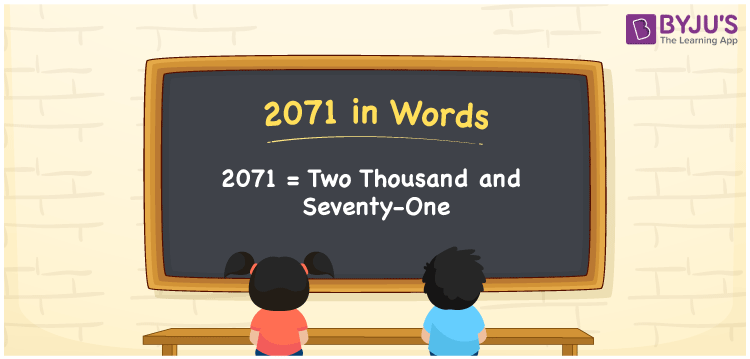# 2071 in Words

We can write 2071 in words as Two thousand and seventy-one. Suppose you have written Rs. 2071 on an expenditure list for a month, then you may spell it as “Two thousand and seventy-one rupees”. Using a place value chart, we can easily derive the word form of the cardinal number 2071.

 2071 in words Two thousand and seventy-one Two thousand and seventy-one in Numbers 2071

## 2071 in English Words

We generally write numbers in words using the English alphabet. Thus, we spell 2071 in English as “Two thousand and seventy-one”.## How to Write 2071 in Words?

In this section, you will learn how to write the number 2071 in words using a place value system.

 Thousands Hundreds Tens Ones 2 0 7 1

Here, ones = 1, tens = 7, hundreds = 0, and thousands = 2.

The above digits can be expanded as follows.

2 × Thousand + 0 × Hundred + 7 × Ten + 1 × One

= 2 × 1000 + 0 × 100 + 7 × 10 + 1 × 1

= 2000 + 70 + 1

= 2000 + 71

= Two thousand + Seventy-one

= Two thousand and seventy-one

Therefore, 2071 in words is written as Two thousand and seventy-one.

2071 is a natural number that precedes 2072 and succeeds 2070.

2071 in words – Two thousand and seventy-one

Is 2071 an even number? – No

Is 2071 an odd number? – Yes

Is 2071 a prime number? – No

Is 2071 a composite number? – Yes

Is 2071 a perfect square number? – No

Is 2071 a perfect cube number? – No

## Frequently Asked Questions on 2071 in Words

Q1

### What is the word form of 2071?

The word form of the numeral 2071 is “Two thousand and seventy-one”.
Q2

### How do you write an amount of Rs. 2071 in words?

We can write an amount of Rs. 2071 in words as “Two thousand and seventy-one rupees”.
Q3

### Write 0.2071 in words.

0.2071 = Two thousand seventy-one ten-thousandths Or 0.2071 = Zero point Two zero seven one